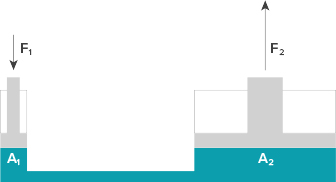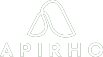Mechanical Engineer Tour Chemical Engineer Tour Software Engineer Tour
Tour Compatibility:
Tours can be viewed well only on laptops/desktops and not on mobile phones currently.
Mechanical Engineer Tour Chemical Engineer Tour Software Engineer Tour
Tour Compatibility:
Tours can be viewed well only on laptops/desktops and not on mobile phones currently.###### Try mechanical engineering design

In this virtual tour you will understand how a mechanical engineer designs products by working on similar engineering assignments.

Note: Assignments are quite simplified compared to real work so that high school students can solve them

# Pre-requisites

Understanding of below concepts and equations (wherever mentioned) is required

View Details

### Pascal’s law

Pascal’s law states that a change in pressure at any point in a fluid in a closed container is transmitted undiminished to all points in the fluid.

By Pascal’s law:

P1 = P2

F1/A1 = F2/A2 (Since pressure equals force per unit area)

(where P = pressure, F = force, A = area)### Friction force

Kinetic friction force between two solid surfaces in contact is the force that opposes the relative motion of those surfaces.

f = μN

(where f = friction force, μ = coefficient of friction, N = normal force)### Torque

Torque is the cross product of position vector (from the axis of rotation to the point where force is applied) and the force vector (which tends to produce rotational motion).

τ = r x F

(where τ = torque vector, r = position vector, F = force vector)### Stress

When a body is subjected to a deforming force, a restoring force is developed in the body. This restoring force per unit area is known as stress.Collapse Details###### Try chemical engineering design

In this virtual tour you will understand how a chemical engineer designs chemical production process by working on similar engineering assignments.

Note: Assignments are quite simplified compared to real work so that high school students can solve them

# Pre-requisites

Understanding of below concepts and equations (wherever mentioned) is required

View Details

### Reaction stoichiometry

Relationship between quantity of reactants and products given by a balanced chemical reaction.

Example:

CH4 + 2O2 -> CO2 + 2H2O

As per above reaction, one mole of CH4 reacts with two moles of O2 to give one mole of CO2 and two moles H2O### Molar mass

Mass of one mole of a substance. It is generally in g/mole.

Example: molar mass of water is 18 g/mole### Distillation

Distillation is separation of components from a mixture based on difference in their boiling points. It involves selective evaporation and condensation.### Volume of right cylinder

V = πr2h

(where V = volume, r = radius, h = height)Collapse Details###### Try software engineering design

In this virtual tour you will understand how a software engineer designs products by working on similar engineering assignments.

Note: Assignments are quite simplified compared to real work so that high school students can solve them